## Mean Curvature

Letandbe the Principal Curvatures, then their Mean(1)

is called the mean curvature. Letandbe the radii corresponding to the Principal Curvatures, then the multiplicative inverse of the mean curvatureis given by the multiplicative inverse of the Harmonic Mean,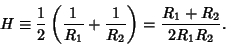(2)

In terms of the Gaussian Curvature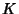,(3)

The mean curvature of a Regular Surface inat a point p is formally defined as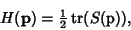(4)

where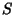is the Shape Operator anddenotes the Trace. For a Monge Patch with,(5)

(Gray 1993, p. 307).

Ifis a Regular Patch, then the mean curvature is given by(6)

where,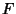, and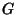are coefficients of the first Fundamental Form and,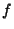, andare coefficients of the second Fundamental Form (Gray 1993, p. 282). It can also be written(7)

Gray (1993, p. 285).

The Gaussian and mean curvature satisfy(8)

with equality only at Umbilic Points, since(9)

If p is a point on a Regular Surface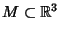andand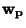are tangent vectors to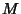at p, then the mean curvature ofat p is related to the Shape Operatorby(10)

Let Z be a nonvanishing Vector Field onwhich is everywhere Perpendicular to, and let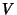and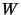be Vector Fields tangent tosuch that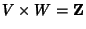, then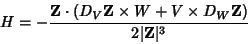(11)

(Gray 1993, pp. 291-292).

Wente (1985, 1986, 1987) found a nonspherical finite surface with constant mean curvature, consisting of a self-intersecting three-lobed toroidal surface. A family of such surfaces exists.

References

Gray, A. The Gaussian and Mean Curvatures.'' §14.5 in Modern Differential Geometry of Curves and Surfaces. Boca Raton, FL: CRC Press, pp. 279-285, 1993.

Isenberg, C. The Science of Soap Films and Soap Bubbles. New York: Dover, p. 108, 1992.

Peterson, I. The Mathematical Tourist: Snapshots of Modern Mathematics. New York: W. H. Freeman, pp. 69-70, 1988.

Wente, H. C. A Counterexample in 3-Space to a Conjecture of H. Hopf.'' In Workshop Bonn 1984, Proceedings of the 25th Mathematical Workshop Held at the Max-Planck Institut für Mathematik, Bonn, June 15-22, 1984 (Ed. F. Hirzebruch, J. Schwermer, and S. Suter). New York: Springer-Verlag, pp. 421-429, 1985.

Wente, H. C. Counterexample to a Conjecture of H. Hopf.'' Pac. J. Math. 121, 193-243, 1986.

Wente, H. C. Immersed Tori of Constant Mean Curvature in.'' In Variational Methods for Free Surface Interfaces, Proceedings of a Conference Held in Menlo Park, CA, Sept. 7-12, 1985 (Ed. P. Concus and R. Finn). New York: Springer-Verlag, pp. 13-24, 1987.